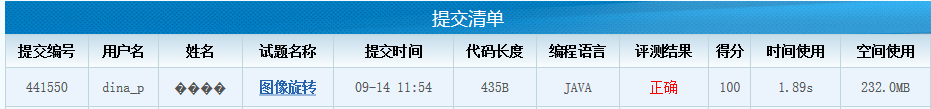• opencv-python-图像旋转-rotation-image
2020-05-12 23:24:37
def rotate_im(image, angle, crop=True):
"""Rotate the image.

Rotate the image such that the rotated image is enclosed inside the tightest
rectangle. The area not occupied by the pixels of the original image is colored
black.

Parameters
----------

image : numpy.ndarray
numpy image

angle : float
angle by which the image is to be rotated
crop: bool
crop the image to the original size

Returns
-------

numpy.ndarray
Rotated Image

"""
# grab the dimensions of the image and then determine the
# centre
(h, w) = image.shape[:2]

(cX, cY) = (w // 2, h // 2)

# grab the rotation matrix (applying the negative of the
# angle to rotate clockwise), then grab the sine and cosine
# (i.e., the rotation components of the matrix)
M = cv2.getRotationMatrix2D((cX, cY), angle, 1.0)
cos = np.abs(M[0, 0])
sin = np.abs(M[0, 1])

# compute the new bounding dimensions of the image
nW = int((h * sin) + (w * cos))
nH = int((h * cos) + (w * sin))

# adjust the rotation matrix to take into account translation
M[0, 2] += (nW / 2) - cX
M[1, 2] += (nH / 2) - cY

# perform the actual rotation and return the image
image = cv2.warpAffine(image, M, (nW, nH))

# if crop the image to the original size
if crop:
(new_h, new_w) = image.shape[:2]
h_delta_half = (new_h - h)/ 2.0
w_delta_half = (new_w - w)/ 2.0

image = image[math.ceil(h_delta_half):new_h - math.floor(h_delta_half),
math.ceil(w_delta_half):new_w -math.floor(w_delta_half),:]

return image


更多相关内容
• 像素阵列操作，例如：图像翻转、旋转、灰度。 先决条件 MATLAB 预实验 读取图像并显示在屏幕上、另存为新图像。 使用 gray_scale() 函数获取强度图像。 教程 << Image Array of Matlab >> Each image include ...
• 问题描述： 参考代码： import java.util.Scanner; /** * * @Author: Dina ... * @Description:图像旋转 * @Score: 100 */ public class _1503_1_ImageRotation { public static void main(String

CCF 历年真题之图像旋转（_1503_1_ImageRotation.java）参考答案

问题描述：

 试题编号： 201503-1 试题名称： 图像旋转 时间限制： 5.0s 内存限制： 256.0MB 问题描述： 问题描述 　　旋转是图像处理的基本操作，在这个问题中，你需要将一个图像逆时针旋转90度。 　　计算机中的图像表示可以用一个矩阵来表示，为了旋转一个图像，只需要将对应的矩阵旋转即可。 输入格式 　　输入的第一行包含两个整数 n, m，分别表示图像矩阵的行数和列数。 　　接下来 n行每行包含 m个整数，表示输入的图像。 输出格式 　　输出 m行，每行包含 n个整数，表示原始矩阵逆时针旋转90度后的矩阵。 样例输入 2 3 1 5 3 3 2 4 样例输出 3 4 5 2 1 3 评测用例规模与约定 　　1 ≤ n, m ≤ 1,000，矩阵中的数都是不超过1000的非负整数。

参考代码：

import java.util.Scanner;
/**
*
* @Author: Dina
* @Time: 2017年9月14日
* @Description:图像旋转
* @Score: 100
*/
public class _1503_1_ImageRotation {

public static void main(String[] args) {
Scanner src=new Scanner(System.in);
int n,m,i,j;
n=src.nextInt();
m=src.nextInt();
int[][] array=new int[n][m];
for(i=0;i<n;i++){
for(j=0;j<m;j++){
array[i][j]=src.nextInt();
}
}
//从最右边的列开始，依次往左开始打印
for(i=0;i<m;i++){
for(j=0;j<n;j++){
System.out.print(array[j][m-i-1]+" ");;
}
System.out.println();
}
src.close();
}

}


提交可通过：展开全文java ccf
• 关于GitHub@ShaneHolmes_OpenCV_image_rotation 源代码： import cv2 img = cv2.imread('C://Users//47463//Desktop//2//cat.jpg') print(img.shape[:2]) height, width = img.shape[:2] M = cv2....

源代码：

import cv2

print(img.shape[:2])

height, width = img.shape[:2]

M = cv2.getRotationMatrix2D((width/2, height/2), 90/2, 1)
img_ro = cv2.warpAffine(img, M, (width, height))

cv2.imshow('rotation', img_ro)

cv2.waitKey()
cv2.destroyAllWindows()• getRotationMatrix2D为计算旋转的规则，第一个参数是旋转的中心轴，此处为图片正中，第二个参数是旋转角度，这里是45度。第三个参数是同向性缩放参数，填写1就可以了
• cv2.warpAffine进行旋转，第一个参数是img，第二个是旋转的规则，第三个是图像的尺寸大小
更多文章：https://blog.csdn.net/qq_33208851/article/details/95237054
展开全文• 图像旋转，能够对抓拍到的图像进行不同角度旋转，正确地描述图像。
• IMAGEROTATION 能够旋转图像。 您可以按度数以任何角度拍摄图像。 这是 matlab IMROTATE 和使用仿射变换矩阵的简单实现。 这段代码没有什么新意。matlab
• 48. 旋转图像（Rotate Image）...图像旋转，实际上是这四个位置上的数对应旋转。 因此，需要找到这四个位置索引的相互关系： matrix[i][j]→matrix[j][n−i−1]→matrix[n−i−1][n−j−1]→matrix[n−j−1][i]matr...

# 题解

## 直接旋转图像旋转，实际上是这四个位置上的数对应旋转。
因此，需要找到这四个位置索引的相互关系：
m a t r i x [ i ] [ j ] → m a t r i x [ j ] [ n − i − 1 ] → m a t r i x [ n − i − 1 ] [ n − j − 1 ] → m a t r i x [ n − j − 1 ] [ i ] matrix[i][j] \to matrix[j][n-i-1]\to matrix[n-i-1][n-j-1]\to matrix[n-j-1][i]
注意两个边界条件
行只需遍历一半 ， [ 0 , n / / 2 ) [0,n//2)
列需要在 [ i , n − i − 1 ) [i,n-i-1)

### 复杂度分析

• 时间复杂度： O ( n 2 ) O\left(n^{2}\right)
• 空间复杂度： O ( 1 ) O(1)

### Python

class Solution:
def rotate(self, matrix: List[List[int]]) -> None:
"""
Do not return anything, modify matrix in-place instead.
"""
n = len(matrix)
for i in range(n//2):
for j in range(i, n - i - 1):
matrix[i][j],matrix[j][n-i-1],matrix[n-i-1][n-j-1],matrix[n-j-1][i] = \
matrix[n-j-1][i], matrix[i][j],matrix[j][n-i-1],matrix[n-i-1][n-j-1]


## 转置矩阵的性质转置后：每一行逆序：逆置边界条件

列需要在 [ i , n ) [i,n)

### 复杂度分析

• 时间复杂度： O ( n 2 ) O\left(n^{2}\right)
• 空间复杂度： O ( 1 ) O(1)

### Python

class Solution:
def rotate(self, matrix: List[List[int]]) -> None:
"""
Do not return anything, modify matrix in-place instead.
"""
n=len(matrix)
for i in range(n):
for j in range(i,n):
matrix[i][j],matrix[j][i]=matrix[j][i],matrix[i][j]
for i in range(n):
matrix[i].reverse()


### Java(待完成)

展开全文leetcode 算法
• 图像旋转样本 iOS 图像视图旋转的示例项目（为 iOS 8 和 Xcode 6.3 构建的项目） 基于的堆栈溢出问题 要检查的相关类是 。 在其中，您将找到示例应用程序 UI 的开始/停止按钮操作： - (IBAction)onButtonPress:...
• 图像顺时针旋转 90 度。说明：你必须原地旋转图像，这意味着你需要直接修改输入的二维矩阵。请不要使用另一个矩阵来旋转图像；示例 1:给定 matrix = [ [1,2,3], [4,5,6], [7,8,9] ], 原地旋转输入矩阵，使其...LeetCode 算法 Java
• 旋转图像(Rotate Image) 文章目录Leetcode算法Java全解答--48.旋转图像(Rotate Image)题目想法结果总结代码我的答案大佬们的答案测试用例其他 题目 给定一个 n × n 的二维矩阵表示一个图像。 将图像顺时针旋转 90 ...leetcode java
• import cv2import numpy as npimport os, shutildef rotate_bound(image, angle):# grab the dimensions of the image and then determine the# center(h, w) = image.shape[:2](cX, cY) = (w // 2, h // 2)# grab t...
• 图像旋转测试 此自述文件概述了在此 Ember 应用程序上进行协作的详细信息。 这个应用程序的简短介绍可以很容易地转到这里。 先决条件 您将需要在您的计算机上正确安装以下东西。 （带有 NPM） 安装 git clone 这个...JavaScript
• 因此，您将拥有：phpBB / ext / w3all / w3imagerotation 将ACP选项“重新编译过时的样式组件”设置为yes 转到“ ACP”>“自定义”>“扩展”，并启用“附件图像旋转”扩展 测试事情可能会做一个测试帖子 如果一切...
• matlab开发-ImageRotation。基于仿射变换矩阵，图像旋转能够旋转图像。
• 第一个元素对应着上文输入的点云在image_2的二维图像，第二个元素的image_shape是对应的图像大小. calib dict{rect,Trv2c,P2} 三维和二维的变换矩阵 cam dict{annotation{boxes(nadarry[n,4])，...
• 基于FPGA全流水线的高质量图像旋转，汤功超，颜露新，本文着重于低时延和减小存储消耗的FPGA全流水线方法作图像旋转。我们使用的方法是基于三通道算法和三次卷积插值。 由于在图像剪切�
• 一个基于EXIF方向旋转JPEG图像的节点模块。 缩略图太大 变更日志 执照 贡献 学分 它有什么作用 此模块根据其EXIF标签将正确的方向应用于JPEG图像。 更准确地说，它是： 旋转像素 旋转缩略图（如果有的话） 在...
• 公司最近的一个项目，其中...图像旋转 python版本： python3 用到的包：from PIL import Image Image下载： pip install pillow # 打开图像 im = Image.open(r'img/1.jpg') # 图像旋转，两种方法： new_img1 = im....python图像处理
• 国际图像处理会议（ICIP 2016），第 3548-3552 页，2016 年。 Bib:@inproceedings{hu2016completed, title={Completed local Derivative Pattern for rotation invariant texture classification}, author={Hu, ...
• 7、订阅/pose_graph/match_points，执行relocalization_callback ros::Subscriber sub_relo_points = n.subscribe("/pose_graph/match_points", 2000, relocalization_callback); 8、创建VIO主线程process()(VINS...
• 3D图像旋转简单的ITK Python(3D Image Rotation Simple ITK Python)我正在尝试使用Simple ITK旋转3D图像。 这是我的代码： imagetoresize是原始图像。 图像大小为（512,512,149）targetimage = imagetoresizeorigin ...
• 在进行图像随机旋转的同时，相应的label值坐标也要进行相应的旋转。 如人脸关键点检测中人脸对应的关键点坐标，物体检测任务中的物体所在box坐标。 （1）.原理-------坐标旋转变换公式 原理参照博客： ...python
• 图像增强 train_datagen = ImageDataGenerator(rescale=1./255) 之前学的图像生成器就有图像增强的功能，上面代码中已经完成了一点图像增强的功能----图像加载的时候就可以完成重新缩放 train_datagen = ...python 计算机视觉
• 1.1 效果展示 图像旋转 二、图像旋转 图像旋转顾名思义就是将图像按照一个对称点进行某个度数的旋转，可以使顺时针，也可以是逆时针，下面来看看实战吧。 2.1 原始图像 2.2 代码实践 # -*- coding: utf-8 -*- # ...
• 其中，(tx,ty)表示平移量，而参数ai则反映了图像旋转，缩放等变化。将参数tx,ty,ai(i=1~4)计算出，即可得到两幅图形的坐标变换关系。 ２）RRPN中用到的变换举例： a）平移变换（Translation) 将每一点移动...
• Qt绘制和自旋转源码，主要是一个简单的小程序，能够使用两种方法旋转一个输入图像；包括了qt的绘制和图像的矩阵变换
• 大多数基于样本块的图像修复算法通过平移来寻找最优匹配块, 当破损区域与信息区域存在旋转或尺度缩放时, 这些算法则无法对图像进行有效修复。针对这一问题, 提出一种基于样本块的旋转及缩放图像修复算法。利用局部...
• 在仅估计鱼眼图像焦距和主点的情况下，该方法先将鱼眼图像上的特征点投影到球面透视图像上，通过估计球面透视图像之间的旋转矩阵和平移向量来建立鱼眼图像特征点的对应区域，并利用球面图像上反投影点误差和的最小化...
• jqueryrotate 在此处查看文档/示例页面： :
•c++ leetcode...